Index: N-Z

 HOME >> Index >> Index: N-Z

This Index page contains all of the commands beginning with a letter from N to Z. All optional arguments that you can put in commands are enclosed in brackets ([]). For details about a command, click on the link(s) to the tutorial(s) where the command is discussed.

Normal
The Normal notation mode is the usual way that we express numbers, with digits to the left and right of the decimal, as in 1357.24. If the answer that you want to display cannot be displayed in 10 digits (or the absolute value of a number less than 0.001), the calculator will express the answer in scientific notation. So if you have a number like 10 billion, it won't display 10,000,000,000, but 1E10. To find the Normal command press the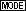key and then.

 Discussed In: Tutorial 25

Not Equal To ()
A test command that checks to see if valueAvalueB (if valueA is not equal to valueB). This command is used a lot with conditionals. You can find the not equal to command by pressing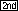, then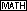[TEST], and then scrolling down to 2:.

 Discussed In: Tutorial 4

Output(
Displays text or value on the current home screen beginning at row (1 through 8) and column (1 through 16), overwriting any existing characters. Output(row,column,"text") You can find it by pressing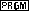and then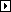and you should be in the I/O section. Scroll down to 6:Output(.

 Discussed In: Tutorial 2

Pause
Pause, when it's just by itself, suspends program execution until the user presses. However, when it's followed by a value, it displays that value and then suspends program execution until user presses. Whatever screen that was last displayed, whether it's the home screen or graph screen, will be displayed untilis pressed. You can tell a program has been paused because of the dashed line in the upper right hand corner of the screen. A solid line means the calculator is thinking, but a dashed line means that the program is paused. To access Pause pressand scroll down to 8:Pause.

 Discussed In: Tutorial 8

Picture Variables
Picture Variables are the variables in which pictures are stored into. Pic1 through Pic9, and Pic0 These variables are not like normal user variables. You cannot store a picture into these variables by using "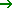" and one of the variables. You have to use the command StorePic to do that. Also, you cannot just display a picture by doing Disp Picn. You have to use the command RecallPic for that. The only time that you actually use these picture variables is when you want to send them to someone else's calculator. To find the list of picture variables, press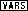and scroll down to 4:Picture... Then choose from the 10 picture variables listed.

 Discussed In: Tutorial 19

Prompt
Prompt displays each variable, on separate lines, each followed by =?. At each prompt, enter a value for each variable, and then press. The values are stored, and the program resumes execution. If you want to prompt more than one variable, you must separate the variables with commas. Y= functions are not valid with Prompt. When a program prompts for input of a list you must surround your answer with {}. When it prompts you to input a value for a matrix you must surround the numbers with []. Finally, when it prompts you to input a string you must surround the text with "". To access Prompt press,, and then scroll down to 2:Prompt.

 Discussed In: Tutorial 5

Pt-Change(
Pt-Change( toggles or reverses a point at coordinate (x,y). Pt-Change(x,y) If you are using Pt-Change along with text you should set the window variables to be compatible with text. To find Pt-Change(, pressplus[DRAW], and then. Scroll down to 3:Pt-Change(.

 Discussed In: Tutorial 13

Pt-Off(
Pt-Off turns of drawn point at coordinate (x,y). Pt-Off(x,y[,mark]) If you specified mark to turn on a point with Pt-On, you must specify mark when you turn of the point with Pt-Off(. If you are using Pt-Off along with text you should set the window variables to be compatible with text. To find Pt-Off(, pressplus[DRAW], and then. Scroll down to 2:Pt-Off(. mark is optional; it determines the point's appearance; specify 1, 2, 3, where:

 1 = dot; default 2 = box 3 = cross

 Discussed In: Tutorial 13

Pt-On(
Pt-On turns of drawn point at coordinate (x,y). Pt-On(x,y[,mark]) If you are using Pt-On along with text you should set the window variables to be compatible with text. To find Pt-On(, pressplus[DRAW],, and then. mark is optional; it determines the point's appearance; specify 1, 2, 3, where:

 1 = dot; default 2 = box 3 = cross

 Discussed In: Tutorial 13

Pxl-Change(
Pxl-Change( toggles the pixel at (row,column), where row is an integer between 0 and 62 and column is an integer between 0 and 94. Pxl-Change(row,column) To find Pxl-Change( pressand[DRAW], and then press. Scroll down to 6:Pxl-Change(.

 Discussed In: Tutorial 14

Pxl-Off(
Pxl-Off( turns off the pixel at (row,column), where row is an integer between 0 and 62 and column is an integer between 0 and 94. Pxl-Off(row,column) To find Pxl-Off( pressand[DRAW], and then press. Scroll down to 5:Pxl-Off(.

 Discussed In: Tutorial 14

Pxl-On(
Pxl-On( turns on the pixel at (row,column), where row is an integer between 0 and 62 and column is an integer between 0 and 94. Pxl-On(row,column) To find Pxl-On( pressand[DRAW], and then press. Scroll down to 4:Pxl-On(.

 Discussed In: Tutorial 14

pxl-Test(
pxl-Test( returns 1 if the pixel at (row,column) is turned on or 0 if the pixel is turned off on the current graph. row is an integer between 0 and 62 and column is an integer between 0 and 94. pxl-Test(row,column) To find pxl-Test( pressand[DRAW], and then press. Scroll down to 7:pxl-Test(.

 Discussed In: Tutorial 14

The Radian mode inteprets angle values in trigonometric functions as radians and displays answers in radians. So if you put sin(30), it would not interpret it as 30°, but as 30 radians. You generally would not use this command in programs, unless you were making a program that actually deals with trigonometric functions. You can find Radian by pressing,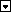twice, and.

 Discussed In: Tutorial 25

randInt(
The command randInt( generates and displays a random integer within a range for a specified number of trials. randInt(lower,upper[,numtrials]) lower is the lower number of the range, and upper is the upper number in the range. You don't have the put the number of trials if you only want one random number. If you put the number of trials, it'll give it to you in the form of a list with {} around the numbers. You can also store the random integer into a user variable. To use this command press, then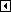, and scroll down to 5:randInt(.

 Discussed In: Tutorial 11

Real
The Real mode is the mode for real numbers. This mode is the mode we usually work in. It does not display complex values unless complex numbers are entered in as input. Calculators are initially set in the Real mode, but once people start getting into the tail end of Algebra they'll mostly likely put their calculators into the a+bi mode. To find Real, press,six times, and.

 Discussed In: Tutorial 25

RecallPic
RecallPic displays the specified picture on the graph screen that was stored in one of the picture variables. RecallPic n To find RecallPic, pressand[DRAW], and then. Scroll down to 2:RecallPic.

 Discussed In: Tutorial 19

Repeat
Repeat repeats the group of commands between the Repeat and End until the condition is true. It is similar to While, but the condition is tested when End is encountered. Therefore, the group of commands is always executed at least once. To access this command pressand then scroll down to 6:Repeat.

 Discussed In: Tutorial 10

round(
round( returns a number, expression, list, or matrix rounded to the specified number of decimal places. The specified number of decimals places is less than or equal to nine. If the number of decimal places is omitted, the value is rounded to the digits that are in it, up to 10 digits. To find round(, pressand. Scroll down to 2:round(.

 Discussed In: Tutorial 30

Sci
The Sci (scientific) notation mode expresses numbers in scientific notation, which has two parts. The significant digits display with one digit to the left of the decimal, and the appropriate power of 10 displays to the right of the E (x10), as in 1.234567E8. Scientific notation will only display on the home screen. All answers displayed on the graph screen are displayed in the Normal mode. To find the Sci command press,and then.

 Discussed In: Tutorial 25

Shade( shades in between a defined upper and lower bound with different patterns and patres. Shade(lowerfunc,upperfunc[,Xleft,Xright,pattern,patres]) lowerfunc is the lower function and upperfunc is the upper function, and they are both the type of functions that you would put in Y=. Shade draws the lower function and upper function in terms of X on the current graph, and shades the area that is specifically above the lower function and below the upper function. Only areas where the lower function is less than the upper function are shaded. Xleft and Xright, if included, specify left and right boundaries for the shading. Xleft and Xright must be numbers between Xmin and Xmax, which are the defaults.

pattern specifies one of four shading patterns:
 pattern = 1 vertical (default) pattern = 2 horizontal pattern = 3 negative-slope 45 ° pattern = 4 positive-slope 45 °

patres specifies one of eight shading resolutions:
 patres = 1 shades every pixel (default) patres = 2 shades every second pixel patres = 3 shades every third pixel patres = 4 shades every fourth pixel patres = 5 shades every fifth pixel patres = 6 shades every sixth pixel patres = 7 shades every seventh pixel patres = 8 shades every eighth pixel

If you want to use shading with points, lines, or circles you will have to set the window variables because they use the coordinate plane. However, if you are using shading with functions, you may have to use different window variable settings. To find Shade(, pressand[DRAW]. Scroll down to 7:Shade(.

 Discussed In: Tutorial 17

Shells
Shells act like a Windows for the calculator and are made using Assembly language. They display all compatible games in a list, so you can easily choose which game or program you'd like to play. They also display how much memory you have left and how big the program you've highlighted is. The three shells that I'll discuss are AShell (the one I mainly use), SOS (I've tried once and very similar to AShell), and TI-Explorer (I have never used this before and just recently heard of it). You can make any BASIC program compatible for these assembly shells by putting a : (colon) at the beginning of your program.

• TI-Explorer is a powerful shell, which works for only the TI-83 and on the screen looks a lot like Windows Explorer. The good thing about this shell is that it runs AShell, SOS, and Ion programs. To download it, click here.
• Ion is the newest of the assembly shells and works for both th TI-83 and TI-83 Plus. Games that are Ion-compatible are the only games that can work with either the TI-83 or TI-83 Plus. AShell- or SOS-compatible games will not work on TI-83 Pluses. To download it for TI-83, click here. For the TI-83 Plus, click here.
 Discussed In: Tutorial 1

Stop
Stop ends program execution and returns to the home screen. You can access Stop by pressingand then scroll down to F:Stop.

 Discussed In: Tutorial 6

Store ()
Stores a value into a variable: valuevariable. To access this command press the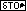button.

 Discussed In: Tutorial 3

StorePic
StorePic stores the current picture that is on the graph screen into one of the picture variables. StorePic n n is a number from 0 to 9. If you were to put 3 for n, then the TI 83/83+ will store the picture to Pic3. To find StorePic, pressand[DRAW], and thenand.

 Discussed In: Tutorial 19

Strings
A string is a type of variable in which you can store text into. A string defines text that is to be displayed in a program and accepts input from the keyboard in a program. Your calculator has 10 string variables: Str0 to Str9. To store text into a string variable: "Put Text Here"Strn. You can also add strings together to make a combined string. "TextA" + " " + "TextB" = "TextA TextB". You can only add strings, you cannot subtract them. You can find the string variables by pressing. Move the cursor down to 7:String... Pressto display the STRING secondary menu. Choose from the 10 string variables.

 Discussed In: Tutorial 3

Tangent(
Tangent( draws a line tangent to expression in terms of X, such as Y1 and X2, at point where X=value. Tangent(expression,value) What you set as your value is the point where the line will touch the function. You can find Tangent( by pressingand[DRAW]. Scroll down to 5:Tangent(.

 Discussed In: Tutorial 18

Text(
Text( writes text on graph beginning at the top-left pixel of the first character at (row,column), where row is an integer between 0 and 57 and column is an integer between 0 and 94. Text(row,column,text1,text2,...,text n). Unlike the Output( command, you can link the texts or values together seperating them by commas. Ex. The value of A is 16. Text(10,10,"I AM ",A," YEARS OLD.") You can find Text( by pressingand then[DRAW]. Scroll down to 0:Text(.

 Discussed In: Tutorial 12

Then

 Discussed In: Tutorial 4

User Variables
A user variable is an alpha character in which you can store a number into. That number can be either a real number (324 or 87) or complex number (i or 5+3i). These are the variables that you will most likely use the most.

 Discussed In: Tutorial 3

Vertical
Vertical draws a vertical line through the x coordinate that travels from the top to the bottom of the screen. Vertical x Because Vertical uses the coordinate plane, you will have to set the window variables. You can find Vertical by pressingand[DRAW]. Scroll down to 4:Vertical.

 Discussed In: Tutorial 15

While
While performs a group of commands while the condition is true. The condition is tested when While is encountered. If the condition is true, the program executes the commands within the While and End. When the condition is false, the program skips the whole While loop and executes the commands following the End command. To access this command pressand then scroll down to 5:While.

 Discussed In: Tutorial 10

Window Variables
The four window variables that are neccessary to change to make points, lines, and circles compatible with graph screen text are: Xmin, Xmax, Ymin, and Ymax. The text will still display if these window variables are not set to what I suggest, but it is easier to align the text with lines, points, and circles with the variables set to what I suggest. There are other window variables such as Xscl, Yscl, and Xres, but they do not affect how the size of the screen. Those three variables are for when you're actually graphing something. To make points, lines, and circles compatible with your text, set the four varibles like this: 0Xmin, 94Xmax, 0Ymax, and -62Ymin. Xmin must be less then Xmax and Ymin must be less than Ymax in order for it to work. You can find all of the window variables by pressing,, and then scrolling down to whichever variable you want.

 Discussed In: Tutorial 13, Tutorial 15, and Tutorial 16

Zoom In
Zoom In magnifies the part of the graph that surrounds the cursor location. To access this command press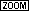and then scroll down to 2:Zoom In.

 Discussed In: Tutorial 26

Zoom Out
Zoom Out displays a greater portion of the graph, centered on the cursor location. To access this command pressand then scroll down to 3:Zoom Out.

 Discussed In: Tutorial 26

ZoomFit
ZoomFit recalculates Ymin and Ymax to include the minimum and maximum Y values, between Xmin and Xmax, of the selected functions and replots the functions. The current values of Xmin and Xmax are not changed. Basically, what is does is fits the entire graph in the current viewing window. To access this command pressand then scroll down to 0:ZoomFit.

 Discussed In: Tutorial 26

ZStandard
ZStandard replots the functions immediately, updating the window variables to the default values. The default values are: Xmin = -10, Xmax = 10, Xscl = 1, Ymin = -10, Ymax = 10, Yscl = 1, and Xres = 1. To access this command pressand then scroll down to 6:ZStandard.

 Discussed In: Tutorial 26

ZTrig
ZTrig replots the functions, updating the window variables to preset values that are appropriate for plotting trigonometric functions. To access this command pressand then scroll down to 7:ZTrig.

 Discussed In: Tutorial 26